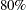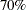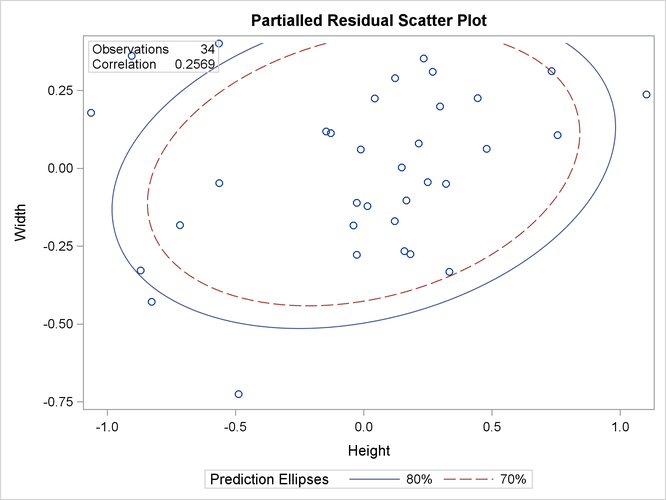### Example 2.9 Computing Partial Correlations

A partial correlation measures the strength of the linear relationship between two variables, while adjusting for the effect of other variables.

The following statements request a partial correlation analysis of variables `Height` and `Width` while adjusting for the variables `Length3` and `Weight`. The latter variables, which are said to be partialled out of the analysis, are specified with the PARTIAL statement.

```ods graphics on;
title 'Fish Measurement Data';
proc corr data=fish1 plots=scatter(alpha=.20 .30);
var Height Width;
partial Length3 Weight3;
run;
ods graphics off;
```

Output 2.9.1 displays descriptive statistics for all the variables. The partial variance and partial standard deviation for the variables in the VAR statement are also displayed.

Output 2.9.1: Descriptive Statistics

 Fish Measurement Data

The CORR Procedure

2 Partial Variables: Length3 Weight3 Height Width

Simple Statistics
Variable N Mean Std Dev Sum Minimum Maximum Partial
Variance
Partial
Std Dev
Length3 34 38.38529 4.21628 1305 30.00000 46.50000
Weight3 34 8.44751 0.97574 287.21524 6.23168 10.00000
Height 34 15.22057 1.98159 517.49950 11.52000 18.95700 0.26607 0.51582
Width 34 5.43805 0.72967 184.89370 4.02000 6.74970 0.07315 0.27047

When you specify a PARTIAL statement, observations with missing values are excluded from the analysis. Output 2.9.2 displays partial correlations for the variables in the VAR statement.

Output 2.9.2: Pearson Partial Correlation Coefficients

Pearson Partial Correlation Coefficients, N = 34
Prob > |r| under H0: Partial Rho=0
Height Width
Height
 1.00000
 0.25692 0.1558
Width
 0.25692 0.1558
 1.00000

The partial correlation between the variables `Height` and `Width` is 0.25692, which is much less than the unpartialled correlation, 0.92632 (in Output 2.9.2). The-value for the partial correlation is 0.1558.

The PLOTS=SCATTER option displays (in Output 2.9.3) a scatter plot of the residuals for the variables `Height` and `Width` after controlling for the effect of variables `Length3` and `Weight`. The ALPHA=.20 .30 suboption requestsandprediction ellipses, respectively.

Output 2.9.3: Partial Residual Scatter PlotIn Output 2.9.3, a standard deviation of `Height` has roughly the same length on the X axis as a standard deviation of `Width` on the Y axis. The major axis length is not significantly larger than the minor axis length, indicating a weak partial correlation between `Height` and `Width`.